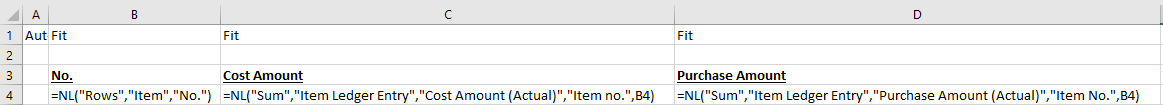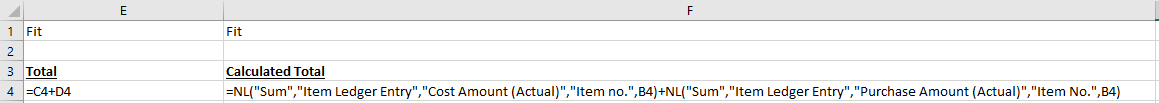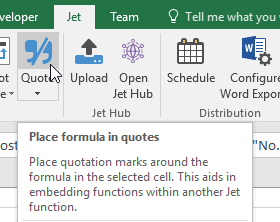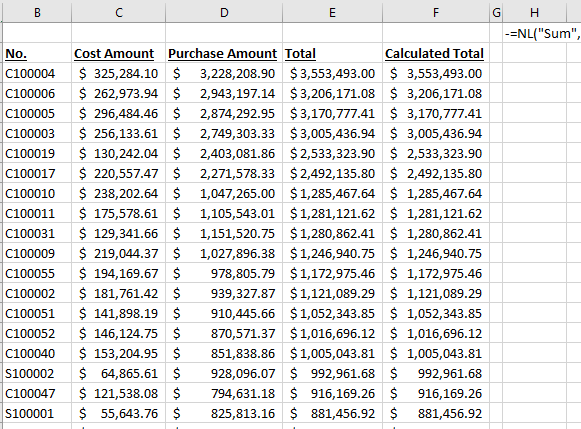Hello,

I'm having trouble with sorting by sum. I have read through a few posts and have nailed down sorting from a separate table but I want to use the sum of multiple tables.

I want a report that produces a list of the top 10 highest costed jobs.

We store the jobs in question in the Service Call table. Invoiced costs are stored in the Contract Ledger Entry table and Committed Costs in the Contract Committed Costs table. Total cost for a job is therefore made of the sum of the two

This would give me the top 10 jobs by invoiced costs

=NL("Rows","Service Call",, "Limit=","10","-=NL(""Sum"",""Contract Ledger Entry"",""Line Amount (LCY)"", ""Contract No."",NF(,""Call No.""))","*")

or this the top 10 jobs by committed cost

=NL("Rows","Service Call",, "Limit=","10","-=NL(""Sum"",""Contract Committed Costs"",""Total Cost (LCY)"", ""Contract No."",NF(,""Call No.""))","*")

but can I sort (the "sum of invoiced costs" plus the "sum of committed costs") and then limit the result. I want something like

=NL("Rows","Service Call",, "Limit=","10","-(=NL(""Sum"",""Contract Ledger Entry"",""Line Amount (LCY)"", ""Contract No."",NF(,""Call No.""))+NL(""Sum"",""Contract Committed Costs"",""Total Cost (LCY)"", ""Contract No."",NF(,""Call No."")))","*")

There are extra filters but i have excluded these to make showing the principle idea easier.

Any help would be greatly appreciated.

Dan

•Harry Lewis

Hi Dan -

Here is the technique I use.

I've created a rudimentary report using the Item and Item Ledger Entry tables in Dynamics NAV (mainly because I have sample data in those tables).

I list out the No. field from the Item table and then, for each item number, the sum of the corresponding Cost Amount (Actual) and Purchase Amount (Actual) fields from the Item Ledger Entry table.Then, I add them together using two different techniques (mostly for ensure that I'm getting the values I expect):I want to use those functions in column F for my sorting.

Using the techniques described in the Sort by Sum article for NAV, I copy the contents of cell F4 to cell H2.

`=NL("Sum","Item Ledger Entry","Cost Amount (Actual)","Item no.",B4)+NL("Sum","Item Ledger Entry","Purchase Amount (Actual),"Item No.",B4)`

Then, since I'm going to reference this function from my NL(Rows) function in cell B4 and I can't have a cell reference itself, I change the references to B4 in my NL(Sum) to NF functions to retrieve the No. field from the Item table:

`=NL("Sum","Item Ledger Entry","Cost Amount (Actual)","Item no.",NF(,"No."))+NL("Sum","Item Ledger Entry","Purchase Amount (Actual),"Item No.",NF(,"No."))`

Next, I use the Quote button on the Jet ribbon...to prepare this function for use as a filter field for my NL(Rows) function:

`="=NL(""Sum"",""Item Ledger Entry"",""Cost Amount (Actual)"",""Item no."",NF(,""No.""))+NL(""Sum"",""Item Ledger Entry"",""Purchase Amount (Actual)"",""Item No."",NF(,""No.""))"`

and then add a minus sign to the start to specify that I want a descending sort:

` ="-=NL(""Sum"",""Item Ledger Entry"",""Cost Amount (Actual)"",""Item no."",NF(,""No.""))+NL(""Sum"",""Item Ledger Entry"",""Purchase Amount (Actual)"",""Item No."",NF(,""No.""))"`

Finally, I add an absolute reference to cell H2 in my NL(Rows) function in cell B4

`=NL("Rows","Item","No.",\$H\$2,"*")`

When I run my report, my results are sorted by the combined sums:It doesn't matter what tables I use (as long as they are related by a field in my base table - in this case, the Item table).

I hope that helps.

•Dan Pope

Hi Harry,

Excellent, that worked a treat. Thanks for you help.